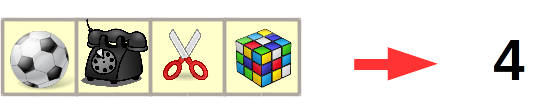# Programming-Idioms# Idiom #117 Get list size

Set n to the number of elements of list x.`n = length x`
```with Ada.Containers.Vectors;
`N : Count_Type := X.Length;`
`size_t n = sizeof(x)/sizeof(*x);`
`(def n (count x))`
`size_t n = x.size();`
`int n = x.Length;`
`using System.Collections.Generic;`
`int n = x.Count;`
`auto n = x.length;`
`import std.range.primitives;`
`n = walkLength(x);`
`int n = x.length;`
`n = Enum.count(x)`
`N = length(X).`
`n = size(x)`
`n := len(x)`
`def n = x.size()`
`var n = x.length;`
`int n = x.size();`
`int n = x.length;`
`val n = x.size`
`(setf n (length x))`
`local n = #x`
`@import Foundation;`
`NSUInteger n=x.count;`
`\$n = count(\$x);`
`n := length(x);`
`\$n = scalar @x;`
```my \$N = @x;
```
`n = len(x)`
`n = x.length`
```let n = x.len();

```
`val n = x.size`
`(define n (length x))`
```Dim x As New List(Of String)(New String() {"one", "two", "three"})
Dim n as Integer = x.Count```

#### Idiom created by

programming-idioms.org